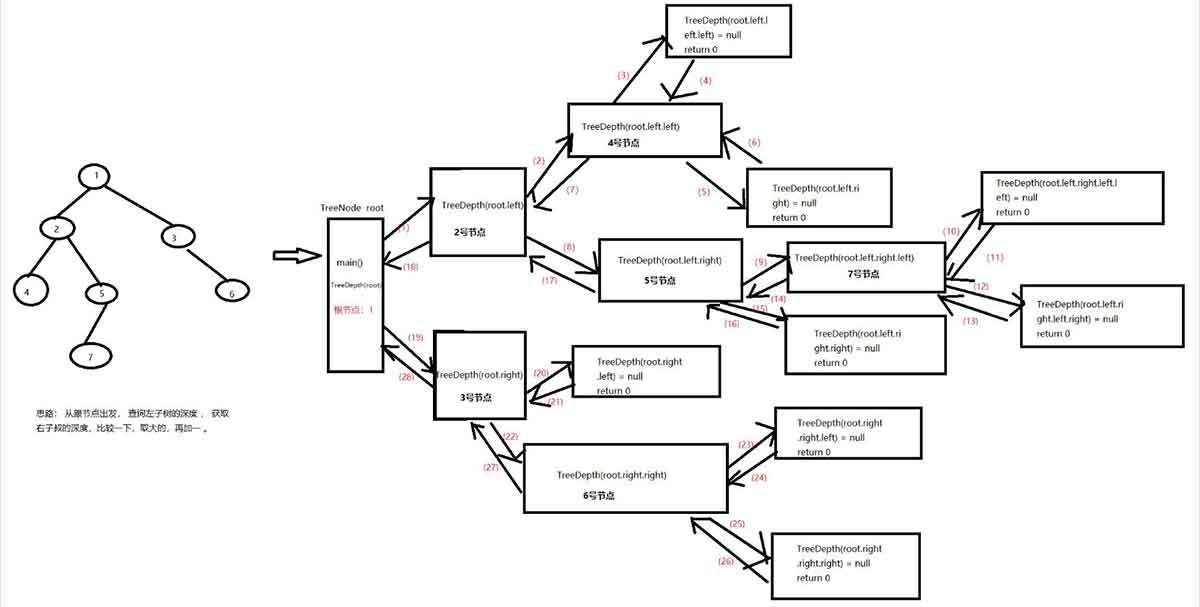# 二叉树的深度怎么算?求二叉树深度的算法(思路和代码实现)

KLQ 2020-04-15 16:23:35 java常见问答 4693

```class Solution
{
public:
int TreeDepth(TreeNode * pRoot)
{
if (!pRoot) return 0;
return max(1 + TreeDepth(pRoot - > left), 1 + TreeDepth(pRoot - > right));
}
};```

```public class Solution
{
public int TreeDepth(TreeNode pRoot)
{
return pRoot == null ? 0 : Math.max(TreeDepth(pRoot.left), TreeDepth(pRoot.right)) + 1;
}
}```

1、边界条件：当前节点下，是否还有子节点，没有返回，有继续递归

2、递归前进段：当前节点下，还有子节点

3、递归返回段：当前节点下，没有子节点```public int TreeDepth1(TreeNode pRoot)
{
if (pRoot == null)
{
return 0;
}
int left = TreeDepth1(pRoot.getLeft());
int right = TreeDepth1(pRoot.getRight());
return Math.max(left, right) + 1;
}```